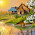### Electric Field Strength or Field Intensity

It is defined as the force experienced by a unit positive charge placed at any point in the electric field. It is represented by symbol E and measured in newton per coulomb.
Suppose a charge of Q coulombs, placed at a point within an electric field, experiences a force of F newtons, then the intensity of the electric field at that point is given by,

Higher the value of E, stronger is the electric field.
Consider a positive charge of Q coulombs  placed in a medium as shown in the Fig.1.

Let a unit positive charge is placed at a distance of d meters from the charge Q.
The field intensity at the point where unit positive charge is placed can be obtained from force experienced by unit positive charge.

The similar concept can be used to obtain the relation between electric field intensity and electric flux density.
1.1   Relation between D and E
Let there be a point charge of ‘Q’ coulombs placed at the centre of the sphere of radius ‘r’ meters. The small positive charge ‘q’ coulombs is placed at a distance ‘r’ from ‘Q’ on the surface of the sphere as shown in Fig.2.

The force experienced on the charge ‘q’ due to ‘Q’ is given by

Electric field strength is given by force per charge.

Substituting in the equation for ‘E’ we get,

#### 1 comment:

1.hi dear its blog very nice and helpful thanks from inverter